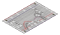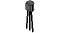﻿ products:nedx:ncir-10[.:oomlout:.]

 NCIR10
 .:Temperature:..:TMP36 Precision Temperature Sensor:.(NEDX) .:Netduino Experimentation Kit:. (NEDX)

What We're Doing
What's the next phenomena we will measure with our Netduino? Temperature. To do this we'll use a rather complicated IC (integrated circuit) hidden in a package identical to our P2N2222AG transistors. It has three pin's, ground, signal and +3.3 volts, and is easy to use. It outputs 10 millivolts per degree centigrade on the signal pin (to allow measuring temperatures below freezing there is a 500 mV offset eg. 25 degrees C = 750 mV, 0 degrees C = 500mV). To convert this from the digital value to degrees, we will use some of the Netduino's maths abilities. Then to display it we'll use one of the IDE's rather powerful features, the debug window. Let's get to it.

This program uses the IDE's debug window to open click Debug > Windows > Output

The TMP36 Datasheet:
http://nedx.org/TMP36

The Circuit
The PartsNCIR-10Breadboard Sheet x12 Pin Headerx4TMP36Temperature Sensor x1WireSchematicResources
http://nedx.org/NBLS10Code (no need to type everything in just)
Download the Code from ( http://nedx.org/CODE10 )
(copy the text and paste it into an empty Netduino Sketch)

//Libraries that our program uses
using System;                               //use base classes
using Microsoft.SPOT;                       //use smart personal objects technology classes
using Microsoft.SPOT.Hardware;              //use hardware related SPOT classes
using SecretLabs.NETMF.Hardware;            //use Secret Labs hardware framework
using SecretLabs.NETMF.Hardware.Netduino;   //use the Netduino specific classes

namespace NCIR10                    /// Define the namespace we are in ///
{
public class Program            /// Define any variables used in the program and subrout
//ines after Main()///
{
static AnalogInput tempSensor = new AnalogInput(Pins.GPIO_PIN_A0);  // defines the t
//emperature sensor as being connected to analog pin 0

public static void Main()   /// The Main loop (run at power up) ///
{
int tempInput = 0;  // defines a variable to store the temperature reading

while (true)           /// Do Forever ///
{
tempInput = tempSensor.Read();    //Read the raw sensor value

float volts = ((float)tempInput / 1024.0f) * 3.3f;  // The read value is fro
//m 0-1023, so this converts it to a % then
// multiplies by 3.3v to
// get the real voltage that was read.
float temp = (volts - 0.5f);                        // The datasheet for the
// sensor indicates there's a 500mV (half a volt)
// offset, this is to al
//low it to read under 0 degrees
temp = temp * 100;                                  // Finally, every 10mV i
//ndicates 1 degree Celsius, so multiply by 100
// to convert the voltag
//e to a reading

Debug.Print(temp.ToString());                       // prints the temperatur
//e reading to the debug window
Thread.Sleep(100);                                  // waits for 100 millise
//conds
}                       /// Close Forever Loop ///
}                           /// Close the Main() Loop ///
}                               /// Close the Program Loop ///
}                                   /// Close the Namespace Loop ///

Not Working? (3 things to try)

Nothing Seems to Happen
This program has no outward indication it is working. To see the results you must open the Netduino IDE's debug window.
(instructions on previous page)

Gibberish is Displayed
This happens because the serial monitor is receiving data at a different speed than expected. To fix this, click the pull-down box that reads "*** baud" and change it to "9600 baud".

Frustration?
Don't worry the Netduino has a great community around it. There's always someone keen to help out on the forums.
http://nedx.org/FORU

Making it Better?

Outputting voltage:
This is a simple matter of changing one line. Our sensor outputs 10mv per degree centigrade so to get voltage we simply debug the volt variable rather than temp. Replace the line:
Debug.Print(temp.ToString());
|
Debug.Print(volts.ToString());

Outputting degrees Fahrenheit:
Again this is a simple change requiring only maths. To go degrees C ----> degrees F we use the formula:
( F = C * 1.8) + 32 )
temp = (temp*1.8f) + 32f;
after
temp = temp * 100;